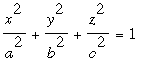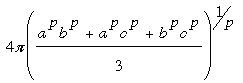SEARCH HOMEMath Central Quandaries & QueriesQuestion from paritosh, a parent: how is the surface area of a elliptical dome be calculated when the two diameters are 55 metres and 35 metres and the height of the structure is 13.4 mts?Paritosh,

There is no easily expressible expression for the surface area of an ellipse. There is however an approximation you can use. Suppose the ellipse has equationwhere a, b and c are the three radii. Wikipedia gives Knud Thomsen's formula for the approximate surface area aswhere p is approximately 1.6075.

I hope this helps,
HarleyMath Central is supported by the University of Regina and The Pacific Institute for the Mathematical Sciences.